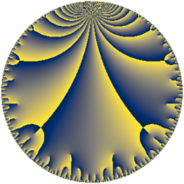# Properties

 Label 43.9.fLevel $43$ Weight $9$ Character orbit 43.f Rep. character $\chi_{43}(2,\cdot)$ Character field $\Q(\zeta_{14})$ Dimension $174$ Newform subspaces $1$ Sturm bound $33$ Trace bound $0$

# Related objects

## Defining parameters

 Level: $$N$$ $$=$$ $$43$$ Weight: $$k$$ $$=$$ $$9$$ Character orbit: $$[\chi]$$ $$=$$ 43.f (of order $$14$$ and degree $$6$$) Character conductor: $$\operatorname{cond}(\chi)$$ $$=$$ $$43$$ Character field: $$\Q(\zeta_{14})$$ Newform subspaces: $$1$$ Sturm bound: $$33$$ Trace bound: $$0$$

## Dimensions

The following table gives the dimensions of various subspaces of $$M_{9}(43, [\chi])$$.

Total New Old
Modular forms 186 186 0
Cusp forms 174 174 0
Eisenstein series 12 12 0

## Trace form

 $$174q - 7q^{2} - 7q^{3} + 4021q^{4} - 7q^{5} + 1780q^{6} - 5383q^{8} + 56490q^{9} + O(q^{10})$$ $$174q - 7q^{2} - 7q^{3} + 4021q^{4} - 7q^{5} + 1780q^{6} - 5383q^{8} + 56490q^{9} - 24989q^{10} - 14864q^{11} - 1799q^{12} + 133078q^{13} - 243363q^{14} - 7457q^{15} - 558511q^{16} + 55162q^{17} - 45934q^{18} + 619745q^{19} + 1207493q^{20} + 307272q^{21} + 432761q^{22} + 473962q^{23} + 179363q^{24} + 2991984q^{25} + 112889q^{26} - 7q^{27} + 458745q^{28} + 1369823q^{29} + 4208148q^{30} + 608540q^{31} - 15074437q^{32} - 10988257q^{33} + 1542905q^{34} - 4078926q^{35} + 53343072q^{36} - 15207265q^{38} - 16862587q^{39} - 537581q^{40} - 852935q^{41} + 2982351q^{43} - 10366284q^{44} + 52423812q^{45} + 51003036q^{46} + 5828578q^{47} - 32078599q^{48} - 145853506q^{49} - 30015937q^{51} + 71975797q^{52} - 7800770q^{53} + 43151245q^{54} - 53485957q^{55} + 25379079q^{56} - 21662515q^{57} - 191329035q^{58} + 69716890q^{59} - 106388277q^{60} - 49131775q^{61} + 67656953q^{62} + 355075q^{63} + 227160853q^{64} - 87064999q^{65} + 146686057q^{66} - 31581292q^{67} + 200919430q^{68} - 30179079q^{69} + 86518670q^{70} - 60255454q^{71} - 438784346q^{72} - 50897868q^{73} + 5087868q^{74} + 206284834q^{75} + 461519674q^{76} + 256281375q^{77} - 182130842q^{78} - 236682026q^{79} - 488977844q^{81} - 226181851q^{82} - 429023996q^{83} - 513228846q^{84} + 336855461q^{86} + 255103986q^{87} + 977894029q^{88} - 258201748q^{89} + 1399550866q^{90} + 246776320q^{91} + 363749100q^{92} - 637129955q^{94} + 60088655q^{95} - 1169196874q^{96} + 218930393q^{97} - 330217825q^{98} + 459482633q^{99} + O(q^{100})$$

## Decomposition of $$S_{9}^{\mathrm{new}}(43, [\chi])$$ into newform subspaces

Label Dim. $$A$$ Field CM Traces $q$-expansion
$$a_2$$ $$a_3$$ $$a_5$$ $$a_7$$
43.9.f.a $$174$$ $$17.517$$ None $$-7$$ $$-7$$ $$-7$$ $$0$$